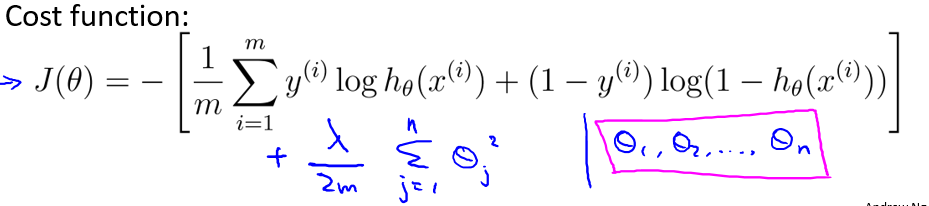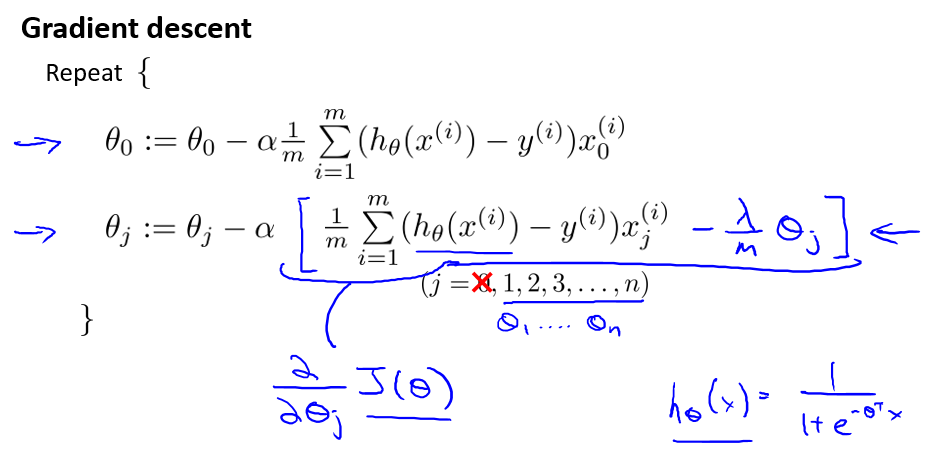# 吴恩达Stanford机器学习公开课（十）笔记

## Lecture 10 - Regularization and model selection.

Posted by Yunlongs on May 11, 2019

# Lecture 10 - Regularization and model selection.

## 1. 交叉验证

1. 对训练数据集$S$使用每个模型$M_i$来训练，得到对应的假设$h_i$
2. 挑选出具有最小训练误差的假设

请注意，这个算法是无效的。因为对于更高阶的多项式，它将能够更好的对训练数据集S进行拟合，这个算法选择出来的模型总是具有高方差，高阶的多项式模型，并不是很好的选择

1. 随机将训练数据集$S$划分为70%的$S_{train}$和30%的$S_{cv}$。其中，$S_{cv}$称作hold-out交叉验证集
2. 使用每个模型$M_i$对$S_{train}$进行训练，得到对应的假设$h_i$
3. 选择并输出具有最小训练误差$\hat{\varepsilon}_ {S_ \mathrm{cv}}(h_{i})$的假设$h_i$

1. 随机将集合$S$均分为k个邻接的子集，将这些子集称为$S_{1}, \dots, S_{k}$
2. 对于每一个模型$M_i$,我们对其做如下的评估：

2.1 For j=1,…,k

在$S_{1} \cup \cdots \cup S_{j-1} \cup S_{j+1} \cup \cdots S_{k}$（除了$S_j$的训练数据集）上对模型$M_i$进行训练，得到对应的假设$h_{i j}$

在$S_j$上对假设$h_{ij}$进行测试，得到$\hat \varepsilon_ {S_j}(h_{ij})$

2.2 计算$\hat \varepsilon_ {S_j}(h_{ij})$的平均值，来得到模型$M_i$的估计泛化误差

3. 选择具有最小估计泛化误差的模型$M_i$,并试用其对整个训练集$S$重新训练。此时输出的x训练参数就是我们最后的算法结果。

## 2.特征选择

1. 初始化$\mathcal{F}=\emptyset$
2. 重复｛

（a）For $i=1, \dots, n$，if $i \notin \mathcal{F},$ let $\mathcal{F}_{i}={\mathcal{F} \cup\lbrace i\rbrace}$。然后使用一些交叉验证方法来评估特征$\mathcal{F}{i}$

(b)设$\mathcal{F}$为步骤(a)中找到的那个最优的特征子集 ｝

3. 选择并输出在整个搜索过程中评估出来的最优特征子集。

## 正则化

### Regularized logistic regression